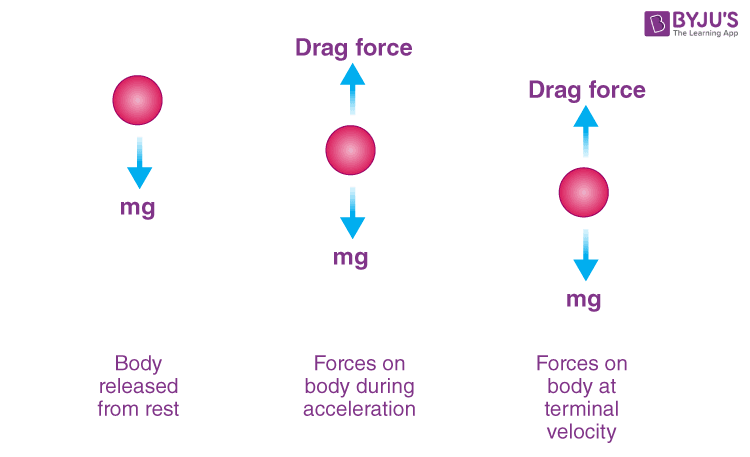Win up to 100% scholarship on Aakash BYJU'S JEE/NEET courses with ABNAT Win up to 100% scholarship on Aakash BYJU'S JEE/NEET courses with ABNAT

# Terminal Velocity

Terminal velocity is the highest velocity that is achieved by an object as it falls through a fluid or a gas. Terminal force occurs when an object is subjected to a resistance that increases with an increase in velocity, and when its resistance equals the driving force. An object that is moving downwards with a higher speed compared to terminal velocity will eventually slow down with terminal velocity.

ExampleFor instance, when a person jumps out of a plane, they tend to gain speed, and they move faster. The reason is the weight force that causes them to accelerate towards the ground. As mentioned earlier, drag force increases with increasing speed. The same holds true for any type of friction. Hence, as the skydiver moves with more speed, they possess more drag force. It continues until the net force of a skydiver is zero, implying that they are no longer accelerating. This states that they have reached terminal velocity. They would continue with this same speed until they open their parachute.

## Mathematical Representation of Terminal Velocity

Terminal Velocity is stated as follows:

$$\begin{array}{l}V_{t}= \sqrt{\frac{2mg}{ρ\ AC_d}}\end{array}$$

• Vt is the terminal velocity.
• Where A represents the projected area of any object.
• ‘m’ represents the mass of the falling object.
• ‘g’ represents acceleration due to gravity.
• ρ is the density of the object.
• Cd is the drag force.

### Derivation of Terminal Velocity

According to the drag equation,

$$\begin{array}{l}F_{net}=ma=mg- \frac{1}{2} ρv^{2}AC_d\end{array}$$

F=0 at Equilibrium,

$$\begin{array}{l}mg- \frac{1}{2}ρv^{2}AC_{d}=0\end{array}$$

By solving this, we get

$$\begin{array}{l}V_{t}= \sqrt{\frac{2mg}{ρ\ AC_{d}}}\end{array}$$

### Calculation of Thermal Velocity

To find the mass of a falling object, it should be measured in the metric system. That is, it should be measured either in kilograms or in grams. Suppose you are using the imperial system; you should make sure of the units.

Determine the acceleration due to gravity. Now, calculate the downward pull of gravity. That is, F=MA. In the case of imperial systems, it is termed l fb of the object, which is commonly termed as weight. It is one of the methods of calculating the thermal velocity.

## Frequently Asked Questions on Terminal Velocity

Q1

### What is terminal velocity?

The maximum velocity attained by an object as it falls through a fluid is called terminal velocity.

Q2

### What happens at the terminal velocity?

As the object attains terminal velocity, it starts moving with a constant speed in a constant direction because the resultant force acting on the object is zero.

Q3

### Does the weight of the body affect the terminal velocity?

The size and shape of the object affect the air drag; this, in turn, will affect the terminal velocity.

Q4

### Is terminal velocity present in a vacuum?

There is no drag force in a vacuum; therefore, terminal velocity does not exist.

Test your Knowledge on Terminal velocity